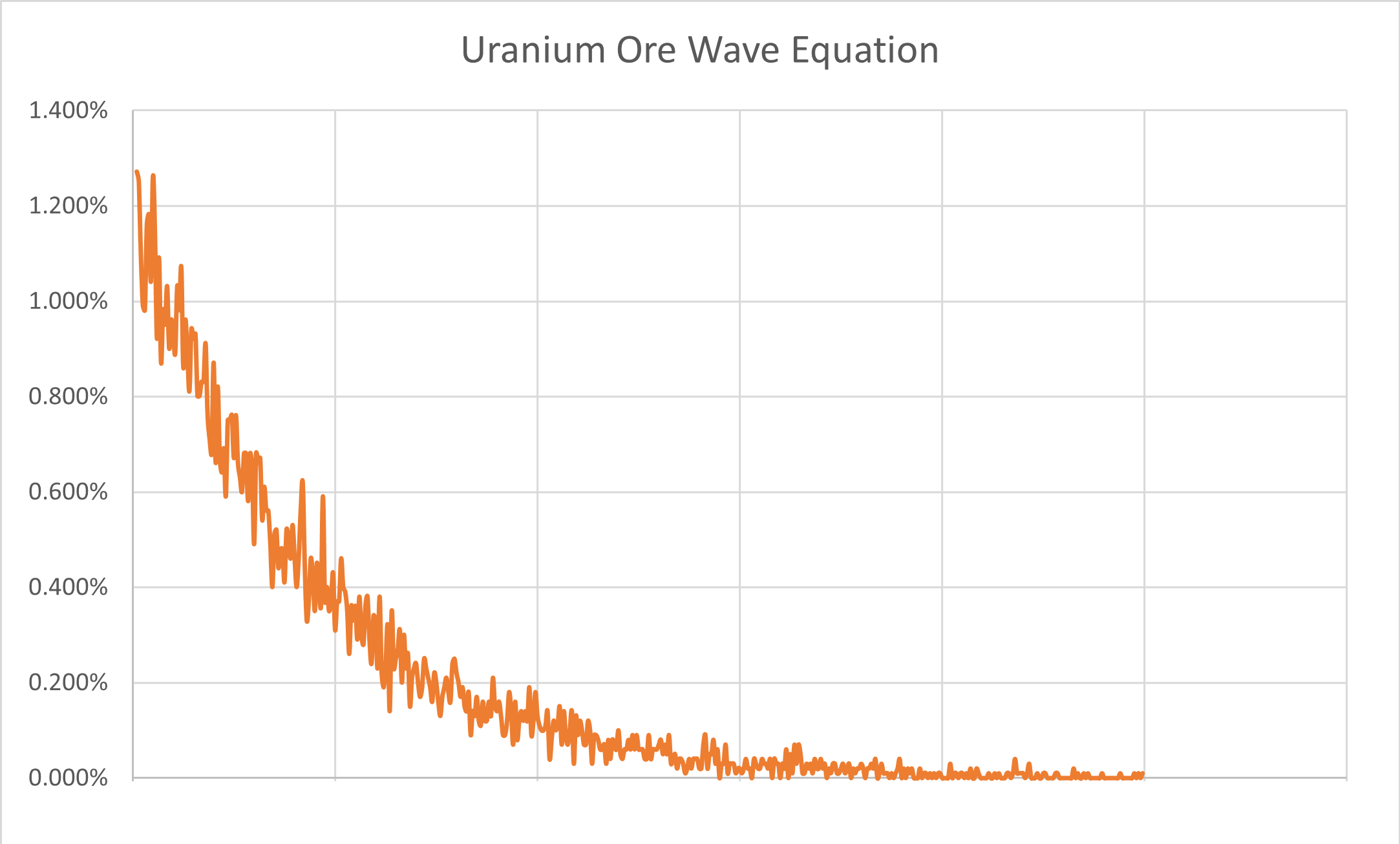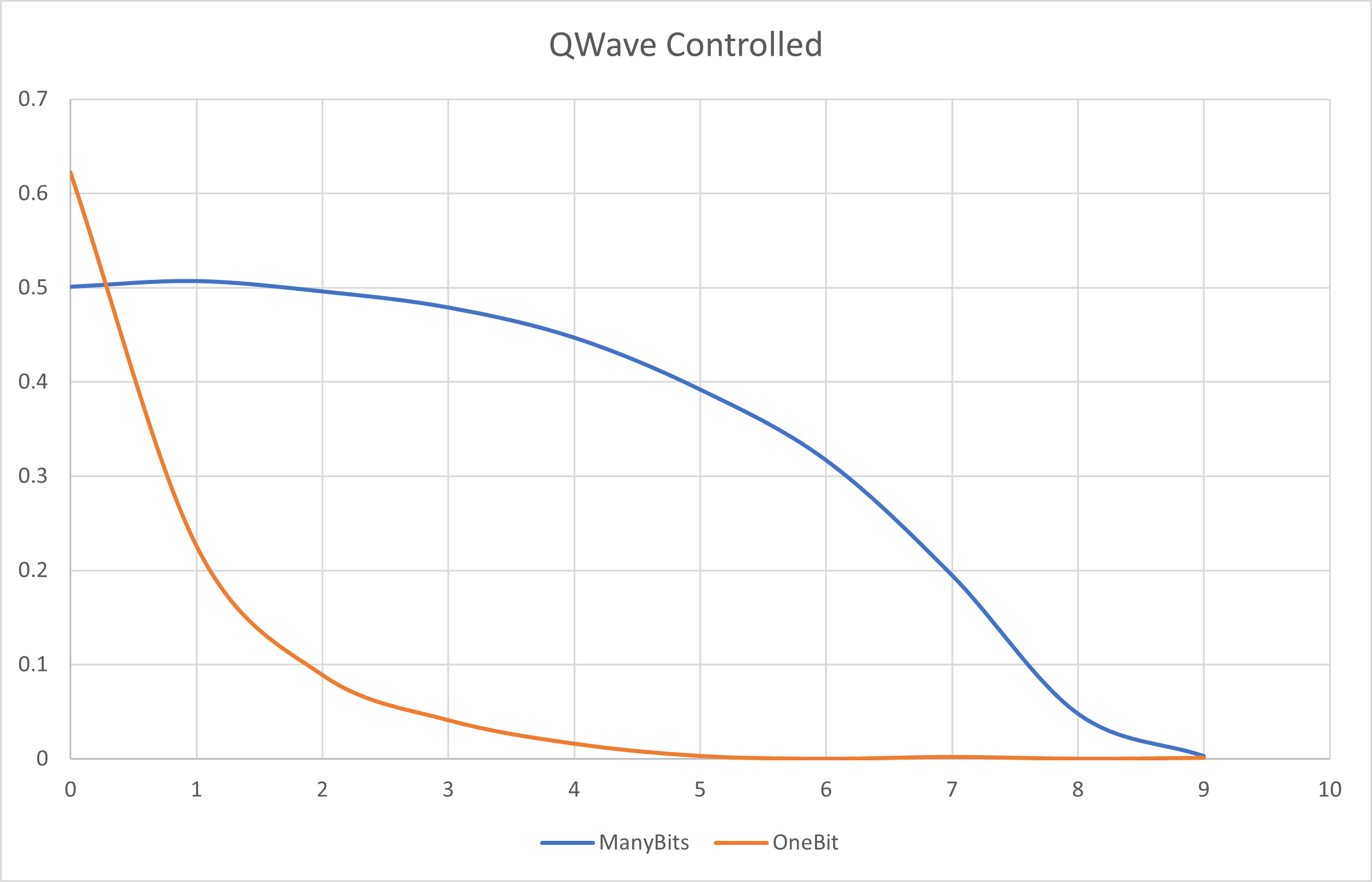# QWave Controlled Gates

A QWave controlled gate encodes a wave function from an outside (real world) quantum state from some quantum system to one or more target qubits. For example, uranium ore emits a radioactive ion (which is a quantum event) at a rate shown in the graph below. The y axis is the probability, and the x axis is the time between emissions. There is a higher probability of emissions occurring rapidly and subsequently a lower probability that there is a significant long (in milliseconds) gap between emissions.## Encoding A Wave Equation To Multiple Qubits

One way (hereby referred to as `ManyBits`) we could encode the wave equation to multiple bits is to simply create a bit representation of the scaled emission time (the x axis above). For example, if we have a four qubit register and we want to apply an emission of 3 milliseconds, we would convert the 3 to binary 1100 (leftmost least significant bit), then apply a chosen gate to the first two qubits in the register. This is what that would look like in a circuit where X is the gate being applied:

``````{
"qubit_count" : 4,
"gates": [
{
"gate_type": "X",
"target_qubits": [0,1,2,3],
"qwave_control": "UraniumOre1:ManyBits"
}
]
}
``````

Another way (hereby referred to as `OneBit`) we could encode the wave equation to multiple bits is to scale the number of bits to the x axis of the graph above and chose only a single bit based upon where the emission time landed. Considering a four qubit register again, a 3-millisecond emission time would convert to binary 1000 - the first bit is the only bit set because this is where a 3 would end up if the graph x axis were divided into four. This is what the same circuit would look like with `OneBit` set:

``````{
"qubit_count" : 4,
"gates": [
{
"gate_type": "X",
"target_qubits": [0,1,2,3],
"qwave_control": "UraniumOre1:OneBit"
}
]
}
``````

To better illustrate the difference between encoding using `ManyBits` vs. `OneBit`, here is how the above wave equation would be encoded on a ten qubit register with these two encoding methods:With the `OneBit` setting, one can see the probability of the given qubit (shown in the x axis) having the chosen gate applied to just it. With `ManyBits`, one can see the probability of any qubit having the chosen gate applied - the first qubit for example would have approximately a 50/50 probability.

## Encoding A Wave Equation to An R-Value

To encode the wave equation into the phase of a single qubit, then the emission event can simply be encoded into the `rvalue` of the appropriate gate. In this case, the emission time is converted to a percentage (the x axis becomse a decimal number from 0 to 1) and then multiplied by the given rvalue. According to the graph above, this would mean a very low probability of the `rvalue` being multiplied by 1.0. This is what a `rvalue` encoded wave equation would look like:

``````{
"qubit_count" : 2,
"gates": [
{
"gate_type": "Rx",
"target_qubits": ,
"rvalue": 6.2831853,
"qwave_control": "UraniumOre1:RValue"
},
{
"gate_type": "Ry",
"target_qubits": ,
"rvalue": 6.2831853,
"qwave_control": "UraniumOre1:RValue"
}
]
}
``````

## Entanglement?

Note that the next two sections are purely theoretical and should be prefaced with "arguably"...

### ManyBits Entanglement

When a qubit register is encoded using the `ManyBits` method, then the entire register is immediately entangled since a single quantum state was used to set every qubit. Any (or all) qubits measured would have to collapse in a way that yields the bits within the original quantum state.

### Temporal Entanglement

In the last example above showing `rvalue` encoding, notice that two quantum states are used one after another. This directly corresponds to two emissions and given the quantum state source (`UraniumOre1`) is the same, then these emissions are potentially entangled across time.